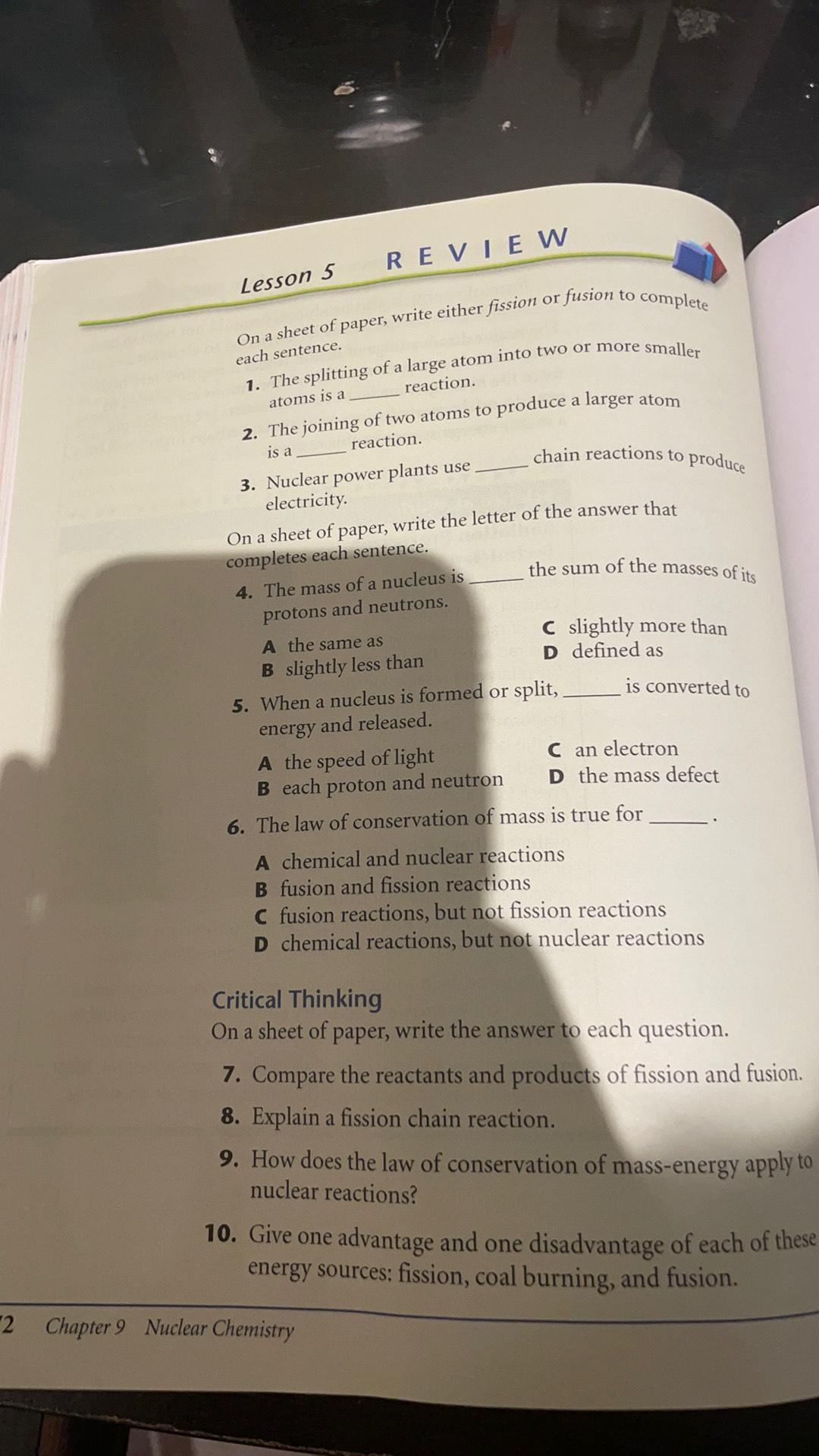Question:

# REVIEW Lesson 5 On a sheet of paper, write either fission or fusion to complete each sentence. 1. The splitting of a large atomREVIEW Lesson 5 On a sheet of paper, write either fission or fusion to complete each sentence. 1. The splitting of a large atom into two or more smaller chain reactions to produce the sum of the masses of its atoms is a reaction. 2. The joining of two atoms to produce a larger atom is a reaction. 3. Nuclear power plants use. electricity. On a sheet of paper, write the letter of the answer that completes each sentence. 4. The mass of a nucleus is protons and neutrons. A the same as B slightly less than 5. When a nucleus is formed or split, energy and released. A the speed of light B each proton and neutron C slightly more than D defined as is converted to C an electron D the mass defect 6. The law of conservation of mass is true for A chemical and nuclear reactions B fusion and fission reactions C fusion reactions, but not fission reactions D chemical reactions, but not nuclear reactions Critical Thinking On a sheet of paper, write the answer to each question. 7. Compare the reactants and products of fission and fusion. 8. Explain a fission chain reaction. 9. How does the law of conservation of mass-energy apply to nuclear reactions? 10. Give one advantage and one disadvantage of each of these energy sources; fission, coal burning, and fusion. 2 Chapter 9 Nuclear Chemistry